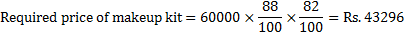# Profit and Loss for IBPS PO : Set 4

Q.1) In a scheme, a box of sweet with MRP Rs.50 is available for Rs.45. If it still gives a profit of 20% to the sweet seller, then what is the cost price of the sweet box?

a) Rs.41.50

b) Rs.39.50

c) Rs.38

d) Rs.37.50

e) None of these

d

Cost price=45/120×100=Rs.37.50

Q.2) A dishonest seller uses 850 gm in place of 1 kg to sell his goods. Find his actual % profit/loss when he sells his article on 10% loss on cost price?

a) 5.88% loss

b) 4.26% loss

c) 5.88% profit

d) 5.25% profit

e) None of these

c

Let cost price of 1 gm be Rs.1

Cost price of 850 gm is Rs.850.

Profit=1000-850=150

Loss on cost price=1000×0.1=100

Total profit=150-100=50

% profit=(150-100)/850×100=5.88%

Q.3) On selling 12 balloon at Rs.900, there is a profit equal to the cost price of 3 balloon. What is the cost price of a balloon?

a) Rs.60

b) Rs.55

c) Rs.80

d) Rs.65

e) Rs.75

a

Let cost price of a balloon be x.

Cost price of 12 balloon=12x

900-12x=3x

15x=900

x=60

Q.4) If percentage of discount offered on marked price of an article is twice the profit percentage and if the selling price of an article is thrice the discount offered, then find the ratio of the discount offered to the cost price.

a) 8:9

b) 3: 8

c) 3: 4

d) 8: 3

e) None of these

b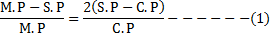S.P=3(M.P-S.P)

3 M.P=4 S.P

Substitute in (1)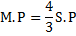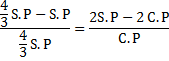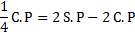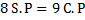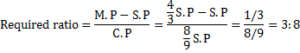Q.5) A wholesale dealer allows a discount of 25% on the price tag to the retailer. The retailer allows 15% discount to the customer on the price tag. If the customer pays Rs.170 for an article, what profit is made by the retailer on it?

a) Rs.10

b) Rs.15

c) Rs.25

d) Rs.20

e) Rs.30

d

Let wholesaler dealers price tag = 100%,

Retailer’s C.P = 75%

If S.P of retailer= 85%

cost price of retailer=170/85×75=150

profit=170-150=Rs.20

Q.6) A quantity of wheat is sold at Rs. 15.25 per kg. The total gain by selling the wheat at this rate is Rs.61. If 20% profit made on this transaction, then find the quantity of wheat being sold?

a) 18 kg

b) 15 kg

c) 22 kg

d) 24 kg

e) None of these

d

Total selling price of the wheat=61/20×120=366

Required quantity of wheat=366/15.25=24 kg

Q.7) If Raj sold his car to Ram at 32% profit and Ram sold to Ravi at 40% profit, then what is the profit in the total transaction? (approximately)

a) 80%

b) 85%

c) 75%

d) 87%

e) 82%

b

Let cost price of Raj be x.

Selling price of Ram=(x×132×140)/10000=1.848 x

total profit in entire transaction=(1.848x-x)/x×100=84.8~85%

Q.8) Vijay marked the price of the motor bike 55% above the cost price and offers 35% discount on the marked price, thereby gaining Rs.375.what is the selling price of the motor bike if he wants to make 20% profit?

a) Rs.50000

b) Rs.60000

c) Rs.55000

d) Rs.65000

e) None of these

b

Profit=S.P-C.P

S.P=155/100×65/100×C.P=1.0075 C.P

1.0075 C.P-C.P=375

0.0075 C.P=375

C.P=375/0.0075=50000

Required selling price=50000×120/100=60000

Q.9) If a company marks the price of the car as Rs.300000 and gives a discount of 22% on auxiliary units fitted to car, Rs.250000 and 15% on the marked price, then what is the selling price of the car?

a) Rs.480000

b) Rs.550000

c) Rs.510000

d) Rs.450000

e) None of these

d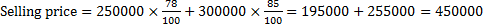Q.10) Geetha purchased a makeup kit with an additional 12% discount on the reduced price after deducting 18% on the labelled price. If the labelled price was Rs.60000, at what price did she purchase the makeup kit?

a) Rs.43264

b) Rs.43396

c) Rs.42369

d) Rs.43296

e) None of these